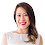### For Genius...

Solve the equation and find the value of  '?'

Check for the right answer below.

Explanation:
Brown Suitcase = 5 (As per the equation 5 + 5 + 5 = 15)

Yellow suitcase = 6 (As per the equation, ((6x2) + 6 + 6) = 24
Note: There is another yellow suitcase behind the first yellow suitcase

Chinese couple = 3 (As per the equation, ((3x6) + (3x6) + (3x6)) = 30
Note: There is a yellow bag (6) in the had of the Chinese women.

There are several answer's and explanations for this question. The above is our explanation. If you have any other answers, please submit in the comments section with explanation.

1.Your explanation to the Chinese couple is wrong. Look again!! The couple explanation is incorrect. Try again.

1.Ur right couple is actually 4

2.3.6+10*4=46 but whats with last couple and tie around his neck??

4.Nope. There are three solutions if all of the images represent natural numbers.
Couple with scarf=y
Couple without scarf=x
Yellow bag=6 (4x6=24)
Brown satchel=5(5x3)=15
Final equation: 6+10x=t (because there are one bag + two satchels multiplied by a couple without the scarf, and t= the unknown)

Couple with scarf > couple without the scarf - y>x

1. x=1, then y=10, then t=16
2. x=2, then y=8, then t=26
3. x=3, then y=6, then t=36
4 x=4, then y=4, but it is impossible because y cannot equal x, as y>x.

IF y and x are natural - we have only three solutions. If y and x are irregular there is an infinite set of solutions

5.Ok, That is only about imagination and logic, so
we have: couple + yellow bag + couple + yellow bag + couple + yellow bag +scarf = 30
1) Simple solution for this equation : (3+6) + (3+6) + (3+3+6) = 30
9 + 9 + 12 = 30, so scarf = 3
2) brown bag = 5, 3*5 = 15
3) yellow bag = 6 4*6 = 24
4) yellow bag + (2 brown bags)*3(no scarf, no yellow bag) = 6 + (5+5)*3 = 36

1.6 + (5+5)*6 = 46

6.I got 36
6+(5×2)×3
6+10×3
6+30=36

7.6 for the scarf

8.9.There are three solutions: 16, 26 and 36, depending on the value of the scarf.

The problem is in the first line. We solve it in two steps:
1. First subtract the 3 yellow bags, 6 each. So 3 'emtpy couples' plus 1 scarf is (30 - 18 = ) 12.
2. Then three options, a, b and c.
a. the 'empty couple' = 1, then the scarf is 9 (total=12). End solution is then 6 + (2x5)*1 = 16.
b. the 'empty couple' = 2, then the scarf is 6 (total=12). End solution is then 6 + (2x5)*2 = 26.
c. the 'empty couple' = 3, then the scarf is 3 (total=12). End solution is then 6 + (2x5)*3 = 36.

There is no option d, because an 'empty couple' can not be more than 3: 3 * 4 = 12; than the scarf would not have any value and would be meaningless.

10.11.12.46 is the correct ans because
Chinese couple=10
Brown suitcase=5
There were 4 bags so,
6+5×8=46 by bodmas

1 bag=6
so,now the ans is

13.(3+6) + (3+6) + (3+3+6) = 30
5+5+5 = 15
(6+6) + 6 + 6 = 24
6 + (5*3) = 21

Scarf : 3
Brown bag : 5
Yellow bag : 6
Couple : 3
Hint : first row last pic has scarf on boy

14.15.It is especially decent, though look into the tips during this home address. Peaches and Screams

16.Hi Buddy,
There is Additional Item & Multiple Item was found :-

1ST ROW - 3RD Couple Man has 1 Black Neck Tie
3RD ROW - 1ST Yellow Bag has (2)
4TH ROW - Brown Suitcase has 2 (see the Double Handle)
4TH ROW - Couple has NO Yellow Bag

Fomula :-
Man Black Neck Tie - 1.5
Brown Suitcase - 5
Yellow Bag - 6
Couple With Yellow Bag & Black Neck Tie - 11
Couple With Yellow Bag - 9.5
Couple Without Yellow Bag - 3.5

17.18.